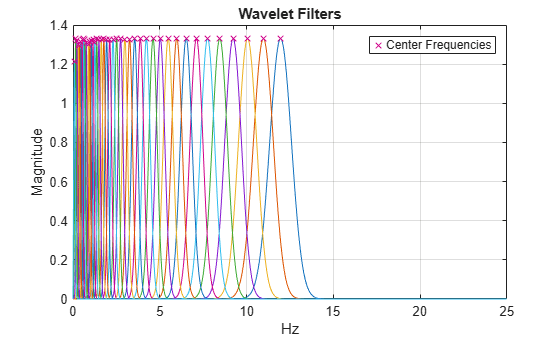# filterbank

Wavelet time scattering filter banks

## Syntax

``filters = filterbank(sf)``
``[filters,f] = filterbank(sf)``
``[filters,f,filterparams] = filterbank(sf)``
``[___] = filterbank(sf,order)``

## Description

````filters = filterbank(sf)` returns the filter banks used in the computation of the scattering coefficients. `filters` is a cell array of structure arrays with norder elements, where norder is the number of scattering orders. The first element of `filters` contains the scaling filter, `phift`, used in the computation of the 0th-order scattering coefficients. Subsequent elements of `filters` contain the wavelet filters, `psift`, and scaling filter, `phift`, for the corresponding filter banks of the scattering decomposition.The precision of `phift` and `psift` depends on the precision specified in the scattering network `sf`.```
````[filters,f] = filterbank(sf)` returns the frequencies corresponding to the DFT bins in the `psift` and `phift` fields of `filters`. If you specify a sampling frequency in the construction of `sf`, `f` is measured in hertz. Otherwise, `f` is measured in cycles/sample.```

example

````[filters,f,filterparams] = filterbank(sf)` returns the filter parameters for each element of `filters`. `filterparams` is a cell array with norder elements. Each element of `filterparams` is a MATLAB® table.```
````[___] = filterbank(sf,order)` returns the filter banks used to compute the specified `order` scattering coefficients. `order` is an integer between 0 and nfilters inclusive, where nfilters is the number of filter banks in the scattering network. These input arguments can be used with any of the output syntaxes shown previously.```

## Examples

collapse all

Create a wavelet time scattering network for a signal sampled at 25 Hz.

`sf = waveletScattering('SamplingFrequency',25)`
```sf = waveletScattering with properties: SignalLength: 1024 InvarianceScale: 20.4800 QualityFactors: [8 1] Boundary: 'periodic' SamplingFrequency: 25 Precision: 'double' OversamplingFactor: 0 OptimizePath: 0 ```

Obtain the filter banks, DFT frequency bins, and filter bank parameters.

`[filters,f,fparams] = filterbank(sf);`

Plot the wavelet filters used in computing the first-order coefficients. Plot the wavelet center frequencies as well.

```coefOrder = 1; wvFilters = filters{coefOrder+1}.psift; wvcenFrq = fparams{coefOrder+1}.omegapsi; plot(f,wvFilters) hold on cf = plot(wvcenFrq,max(wvFilters),'rx'); grid on title('Wavelet Filters') xlabel('Hz') ylabel('Magnitude') legend(cf,'Center Frequencies')```## Input Arguments

collapse all

Wavelet time scattering network, specified as a `waveletScattering` object.

Order of scattering coefficients, specified as a positive integer between 0 and nfilters inclusive, where nfilters is the number of filter banks in the scattering decomposition `sf`.

Data Types: `double`

## Output Arguments

collapse all

Filter banks using in the computation of the scattering coefficients, returned as a cell array of structure arrays. `filters` has norder elements, where norder is the number of scattering orders. The first element of `filters` is a structure with the single field `phift`. `phift` contains the scaling filter used in the computation of the 0th-order scattering coefficients. Subsequent elements of `filters` contain the wavelet filters, `psift`, and the scaling filter, `phift`, for the corresponding filter banks of the scattering network in the structure fields.

The precision of `phift` and `psift` depends on the precision specified in the scattering network `sf`.

Frequencies corresponding to the DFT bins in the `psift` and `phift` fields of `filters`. If you specify a sampling frequency in the construction of `sf`, `f` is measured in hertz. Otherwise, `f` is measured in cycles/sample.

Data Types: `double`

Filter bank parameters for each element of `filters`, returned as a cell array. `filterparams` has norder elements, where norder is the number of scattering orders.

The first element of `filterparams` is a MATLAB table with the following variables:

• `boundary` — The signal extension used in the filters, returned as either `'periodic'` or `'reflection'`.

• `precision` — The precision used in the filters, returned as `'double'` or `'single'`.

• `sigmaphi` — The time standard deviation of the scaling function, returned as a scalar. If you specify a sampling frequency, `sigmaphi` is in seconds. Otherwise, `sigmaphi` is in samples.

• `freqsigmaphi` — The frequency standard deviation of the scaling function, returned as a scalar. If you specify a sampling frequency, `freqsigmaphi` is in hertz. Otherwise, `freqsigmaphi` is in cycles/sample.

• `phiftsupport` — The frequency support of the scaling function, returned as a scalar. If you specify a sampling frequency, `phiftsupport` is in hertz. Otherwise, `phiftsupport` is in cycles/sample.

• `phi3dBbw` — The 3-dB bandwidth of the scaling function, returned as a scalar.

Subsequent elements of `filterparams` include additional variables for the wavelet parameters:

• `J` — The integer number of logarithmically spaced wavelet filters in the scattering filter bank.

• `omegapsi` — The center frequencies for the wavelet filters in descending order (highest to lowest), returned as a vector. The `omegapsi` variable includes the center frequencies for any linearly spaced filters.

• `freqsigmapsi` — The wavelet frequency standard deviations, returned as a vector.

• `timesigmapsi` — The wavelet time standard deviations, returned as a vector.

• `psi3dBbw` — The wavelet 3-dB bandwidths, returned as a vector.

• `psiftsupport` — The wavelet frequency supports, returned as a vector.

## Extended Capabilities

### C/C++ Code GenerationGenerate C and C++ code using MATLAB® Coder™.